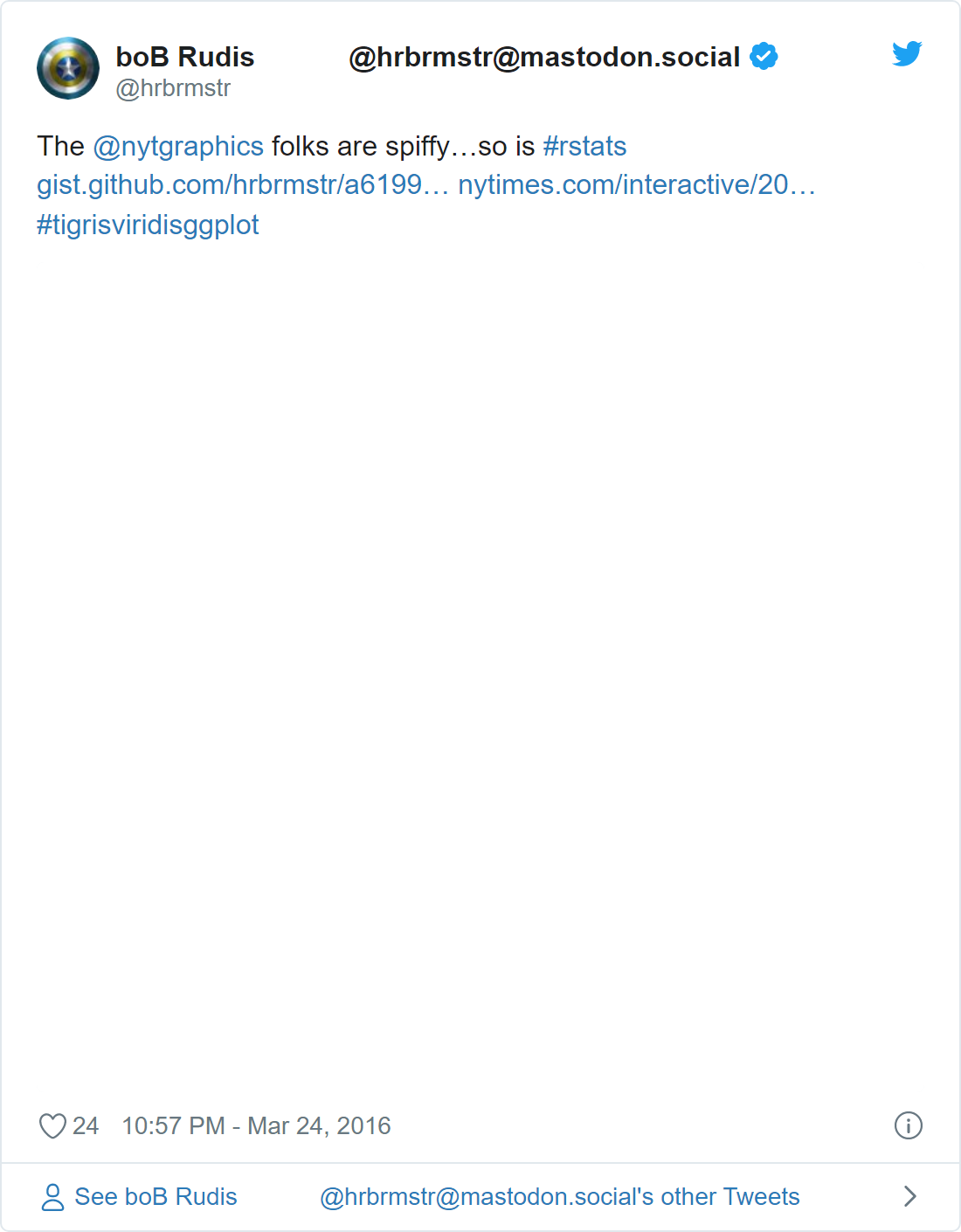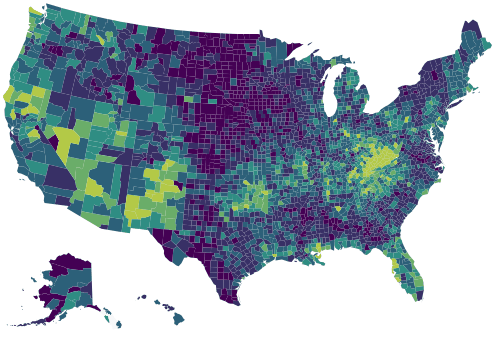We will use the highchart motion plugin with a map.

maps
highcharts
animation
Author

Joshua Kunst Fuentes

Published

April 12, 2016

Time ago hrbrmstr show how to replicate a visualization made by New York Times with R.The result we hope is like this:I really like small multiples and this is a good example of usage. However if the multiples means a lot mini plots maybe you can try add animation.

Let’s start using the script made by Bob:

Code
``````library(jsonlite)
library(dplyr)
library(tidyr)
library(highcharter)

URL <- "http://graphics8.nytimes.com/newsgraphics/2016/01/15/drug-deaths/c23ba79c9c9599a103a8d60e2329be1a9b7d6994/data.json"

data("uscountygeojson")
data("unemployment")

data <-  fromJSON(URL) %>%
as_tibble() %>%
gather(year, value, -fips) %>%
mutate(year = sub("^y", "", year),
value = ifelse(is.na(value), 0, value))

data``````
``````# A tibble: 40,833 × 3
fips  year  value
<chr> <chr> <dbl>
1 01001 2002      1
2 01003 2002      2
3 01005 2002      0
4 01007 2002      1
5 01009 2002      2
6 01011 2002      0
7 01013 2002      0
8 01015 2002      1
9 01017 2002      1
10 01019 2002      1
# … with 40,823 more rows``````

Now we’ll prepare the data as the motion plugin require the data.

Code
``````ds <- data %>%
group_by(fips) %>%
do(item = list(
fips = first(.\$fips),
sequence = .\$value,
value = first(.\$value))) %>%
.\$item

hc <- highchart(type = "map") %>%
data = ds,
name = "drug deaths per 100,000",
mapData = uscountygeojson,
joinBy = "fips",
borderWidth = 0.01
) %>%
hc_colorAxis(stops = color_stops()) %>%
hc_title(text = "How the Epidemic of Drug Overdose Deaths Ripples") %>%
hc_subtitle(text = "Overdose deaths per 100,000") %>%
hc_legend(
layout = "horizontal",
reversed = TRUE,
floating = TRUE,
align = "right"
) %>%
hc_motion(
enabled = TRUE,
axisLabel = "year",
labels = sort(unique(data\$year)),
series = 0,
updateIterval = 50,
magnet = list(
round = "floor",
step = 0.1
)
) %>%
hc_chart(marginBottom  = 100)``````

And the result:

Code
``hc``